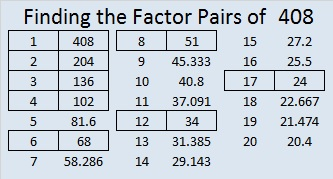# 408 and Level 6

408 is divisible by 8 and so are 1408, 2408, 3408, 4408, . . . . . . and any other number whose last 3 digits are 408. This is true because if the last 3 digits of a number are divisible by 8, the entire number is also!

Also since 4 + 8 = 12, a multiple of 3, we know that 408 is divisible by 3.

408’s factors are listed below the puzzle.Print the puzzles or type the factors on this excel file: 12 Factors 2015-02-23

• 408 is a composite number.
• Prime factorization: 408 = 2 x 2 x 2 x 3 x 17, which can be written 408 = (2^3) x 3 x 17
• The exponents in the prime factorization are 3, 1, and 1. Adding one to each and multiplying we get (3 + 1)(1 + 1)(1 + 1) = 4 x 2 x 2 = 16. Therefore 408 has exactly 16 factors.
• Factors of 408: 1, 2, 3, 4, 6, 8, 12, 17, 24, 34, 51, 68, 102, 136, 204, 408
• Factor pairs: 408 = 1 x 408, 2 x 204, 3 x 136, 4 x 102, 6 x 68, 8 x 51, 12 x 34, or 17 x 24
• Taking the factor pair with the largest square number factor, we get √408 = (√4)(√102) = 2√102 ≈ 20.199This site uses Akismet to reduce spam. Learn how your comment data is processed.